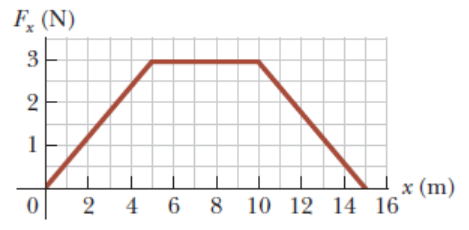# Problem: A particle is subject to a force Fx that varies with position as shown in the following figure. What is the total work done by the force over the distance x = 0 to x = 15.0 m?

###### FREE Expert Solution

Work done from x = 0 to x = 15 is equal to the sum of the areas under the curve. We have two triangles and one rectangle.

92% (260 ratings)###### Problem Details

A particle is subject to a force Fx that varies with position as shown in the following figure. What is the total work done by the force over the distance x = 0 to x = 15.0 m?